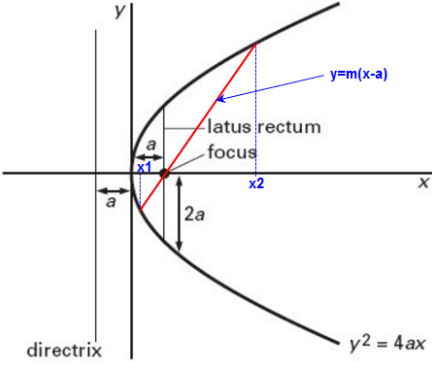# If the vertices of a hyperbola be at  and  and one of its foci be at , then which one of the following points does not lie on this hyperbola ? Option 1)      Option 2)Option 3)Option 4)

Standard equation of parabola -

- whereinGiven, equation of hyperbola

Given

put a = 2

Hence , equation of hyperbola is

Hence  does not lie on hyperbola.

Option 1)

Option 2)

Option 3)

Option 4)

Exams
Articles
Questions